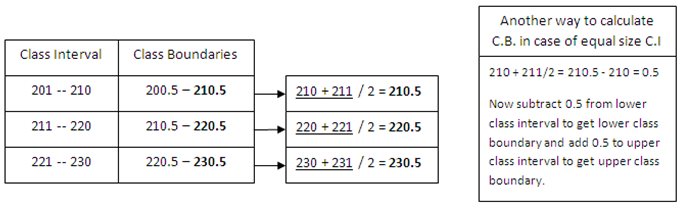Calculation of Class Boundaries and Mid. Class

Calculation of Class Boundaries

Calculation of class boundaries for three class intervals can be illustrated with the help of following figure. The figure shows two methods for calculating the class boundaries. In the first method, class boundaries are calculated by adding the upper class limit of upper class to the lower class limit of next class divided by 2.The second method shows that in case of equal size class interval, calculate class boundaries by adding the upper class limit of upper class to the lower class limit of next class divided by 2.  Now subtract upper class limit of upper class from the number obtained as shown in the figure above. The figure shows that we get 0.5 after this calculation, which can be used to obtain class boundaries by subtracting it from the lower limits of all class intervals and adding it to the upper limits of all class intervals.

Calculation of Middle Class or Mid. Class

Each interval of frequency distribution is represented by the central value of the interval called the mid point of the interval. Mid. class can be calculated by adding the lower and upper class boundaries or by adding the lower and upper class limits and dividing by 2.12 responses to Calculation of Class Boundaries and Mid. Classabid shah says:

this is nice tooAman says:

The first way shown is:
(a) incorrect
(b) horrendously WRONG CALCULATION! It shows, for instance, (201 + 210)/2 = 411/2 = 205.5 –> and how is THAT equal to 210.5???!! MORON!!

People like you are a waste of space. American, I suppose?mbalectures says:

@Aman, Thanks Aman for correction. Although the language used was a bit harsh but we don’t mind because we believe in democracy. If you have found some other mistakes please do tell us in your own style we will never mind. Thanks againammar says:

210-211/2 =0.5 now subtract it to the lower class and add to the upper simpleammar says:

mr aman i think u dint see it that way its 210+210/2=210.5..whch is abslutly crct…Khor Guang Loa says:

The trick is that sometimes the sum of lower and upper class limit may be an even number, and when that sum is divided by two, the resulting answer will a whole number. How can the class boundaries be calculated?Celine Cruz says:

How do u get the class boundaries for decimal numbers? Let say interval is 4.8 – 5.3. I’ve been having difficulty looking for solutions for it.

Thanks a lot in advance. 🙂Red says:

Mr. Aman, if there are problems or if there is a need for correction, just tell it nicely to the one who posted it. Don’t use unnecesesary words.Nithiyaa says:

how to calculate upper boundary for ungroup data according to analysis of businessSheeraz (Mughal's Pakistan) says:

Good work by the person who posted it, . , Mughal got it quite nicely, . ,LCL says:

What is the class mid-value of a class of 0 – 10 using the two methods above (using limits and boundaries)?clement says:

The way Mr Amman type his calculator must be wrong